Mathematical Logic as based on the Theory of Types

BY   BERTRAND  RUSSELL

Published in: American Journal of Mathematics, vol.30(1908), pp. 222-262
Transcribed into hypertext by Burtzev B.I., Jul., 07, 2003
e-mail: bbi-math@narod.ru,   site: http://bbi-math.narod.ru/

VIII.

Descriptive Functions.

EP.The functions hitherto considered have been propositional functions, with the exception of a few particular functions such R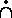S. But the ordinary functions of mathematics, such as x2, sin x, log x, are not propositional. Functions of this kind always mean Уthe term having such-and-such a relation to x.Ф For this reason they may be called descriptive functions, because they describe a certain term by means of its relation to their argument. Thus Уsin π/2Ф describes the number 1; yet propositions in which sin π/2 occurs are not the same as they would be if 1 were substituted. This appears, e.g., from the proposition Уsin π/2 = 1Ф which conveys valuable information, whereas У1 = 1Ф is trivial. Descriptive functions have no meaning by themselves, but only as constituents of propositions; and this applies generally to phrases of the form Уthe term having such-and-such a property.Ф Hence in dealing with such phrases, we must define any proposition in which they occur, not the phrases themselves.24) We are thus led to the following definition, in which У( #x) (φx)Ф is to be read Уthe term x which satisfies φx

ψ { ( #x) (φx)} . = : (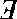b): φx .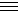x . x = b : ψb    Df.
EQ.This definition states that Уthe term which satisfies φ satisfies ψФ is to mean: УThere is a term b such that φx is true when and only when x is b, and ψb is true.Ф Thus all propositions about Уthe so-and-soФ will be false if there are no so-and-soТs or several so-and-soТs.
ER.The general definition of a descriptive function is

R*y = ( #x) ( xRy)    Df.;

that is, УR*yФ is to mean Уthe term which has the relation R to y.Ф If there are several terms or none having the relation R to y, all propositions about R*y will be false. We put

E ! ( #x) (φx) . = : (b) : φx .x . x = b    Df.

Here УE ! ( #x) (φx)Ф may be read Уthere is such a term as the x which satisfies φx,Ф or Уthe x which satisfies φx exists.Ф We have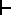: . E ! R*y .: (b) : xRy .x . x = b.
The asterics in R*y may be read of. Thus if R is the relation of father to son, УR*yФ is Уthe father of y.Ф If R is the relation of son to father, all propositions about R*y will be false unless y has one son and no more.
ES.From the above it appears that descriptive functions are obtained from relations. The relations now to be defined are chiefly important on account of the descriptive functions to which they give rise.

Cnv = Q^ P^ { ( xQy .x, y . yPx}    Df.

Here Cnv is short for Уconverse.Ф It is the relation of a relation to its converse; e.g., of greater to less, of parentage to sonship, of preceding to following, etc. We have. Cnv*P = ( #Q) { xQ y .x, y . yPx}.

For a shorter notation, often more convenient, we put

Pº = Cnv*P    Df.

We want next a notation for the class of terms which have the relation R to y. For this purpose, we put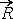= α^ y^ { α = x^ ( xRy) }    Df.,

whence.*y = x^ (xRy).

Similarly we put whence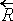= β^ x^ { β = y^ ( xRy) }    Df.,

whence.*x = y^ (xRy).

ET.We want next the domain of R (i.e., the class of terms which have the relation R to something), the converse domain of R (i.e., the class of terms to which something has the relation R), and the field of R, which is the sum of the domain and the converse domain. For this purpose we define the relations of the domain, converse domain, and field, to R. The definitions are:
 D = α^ R^ { α = x^ ((y) . xRy) } Df. Dinv = β^ R^ { β = y^ ((y) . xRy) } Df. C = γ^ R^ { γ = x^ ((y): xRy .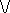. yRx) } Df.
Note that the third of these definitions is only significant when R is what we may call a homogeneous relation; i.e., one in which, if xRy holds, x and y are of the same type. For otherwise, however we may choose x and y, either xRy or yRx will be meaningless. This observation is important in connection with Burali-FortiТs contradiction.
EU.We have, in virtue of the above definitions,. D*R = x^ { (y) . xRy) }. Dinv*R = y^ { (x) . xRy) }. C*R = x^ { (y) : xRy .. yRx) }
the last of these being significant only when R is homogeneous. УD*RФ is read Уthe domain of R;Ф УDinv*RФ is read Уthe converse domain of RФ and УC*RФ is read Уthe field of R.Ф The letter C is chosen as the initial of the word Уcampus.Ф
EV.We want next a notation for the relation, to a class α a contained in the domain of R, of the class of terms to which some member of α has the relation R, and also for the relation, to a class β contained in the converse domain of R, of the class of terms which have the relation R to some member of β. For the second of these we put

Rε = α^ β^ { α = x^ ((y) . y ε β . xRy) }  Df.

So that. Rε*β = x^ { (y) . x ε β . xRy}

Thus if R is the relation of father to son, and β is the class of Etonians, Rε will be the class Уfathers of Etonians,Ф if R is the relation Уless than,Ф and β is the class of proper fractions of the form 1 Ч 2-n for integral values of n, Rε will be the class of fractions less than some fraction of the form 1 Ч 2-n; i.e., Rε will be the class of proper fractions. The other relation mentioned above is (Rº )ε.
EW.We put, as an alternative notation often more convenient,

R**β = Rε  Df.
EX.The relative product of two relations R, S is the relation which holds between x and z whenever there is a term y such that xRy and yRz both hold. The relative product is denoted by R|S. Thus

R|S = x^ z^ { (y) . xRy . yRz }  Df.

We put also

R2 = R|R  Df.

EY.The product and sum of a class of classes are often required. They are defined as follows:
 s*κ = x^ { (α) . α ε κ . x ε α } Df. p*κ = x^ { α ε κ .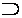α . x ε α } Df.
Similarly for relations we put
 s•*λ = x^ y^ { (R) . R ε λ . xRy } Df. p•*λ = x^ y^ { R ε λ .R . xRy } Df.

EZ.We need a notation for the class whose only member is x. Peano uses ιx, hence we shall use ι*x. Peano showed (what Frege also had emphasized) that this class can not be identified with x. With the usual view of classes, the need for such a distinction remains a mystery; but with the view set forth above, it becomes obvious.
FA.We put

ι = α^ x^ { α = y^ (y =x) }  Df.

whence. ι*x = y^ ( y = x),

and: E ! ιº*α .. ιº*α = (#x) (x ε α);

i.e., if α is a class which has only one member, then ιº is that one member.25)
FB.For the class of classes contained in a given class, we put

Cl*α = β^ ( β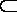α)  Df.

FC.We can now proceed to the consideration of cardinal and ordinal numbers, and of how they are affected by the doctrine of types.

 Notes